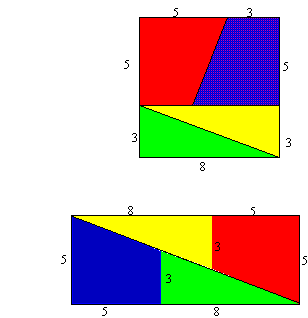#### You may also likeA 1 metre cube has one face on the ground and one face against a wall. A 4 metre ladder leans against the wall and just touches the cube. How high is the top of the ladder above the ground?### From All Corners

Straight lines are drawn from each corner of a square to the mid points of the opposite sides. Express the area of the octagon that is formed at the centre as a fraction of the area of the square.Kyle and his teacher disagree about his test score - who is right?

##### Age 14 to 16Challenge Level

Patrick from Woodbridge School offered the following insight to this problem, as did Chip from King's Ely School.

The difference arises because there is a difference in the line gradient between the trapezium and the triangle. The sloping line of the trapezium has a gradient of $\frac{2}{5} = 0.4$ , whereas the hypotenuse of the triangle has a gradient of $\frac{3}{8} = 0.375$.
This means that the lines made when you join the triangle and trapezium together are not straight, so there is a small amount of space, as shown on my diagram. This space adds up to the extra $1^2$cm.

 An anonymous solver also wrote: The area of the original square was $64$ square units and the area of the rectangle was $65$ square units. What appears to be a diagonal of the rectangle is not in fact a straight line and the extra unit of area comes from the long thin parallelogram in the middle of the rectangle. You can confirm this by making a careful scale drawing for yourself on graph paper. The long thin parallelogram occurs because the slopes of the trapezia are not the same as explained by Patrick and Chip: the slopes of the triangles. For each trapezium the slope is in the ratio of 2 up to 5 across and for each triangle the slope is in the ratio of 3 up to 8 across.Finally, Susie from DEECD Victoria offered an example of other numbers that work (Do they have the same properties as the numbers used for the lengths in the problem?). She said:

Another set of dimensions are $4,7,11$ in the corresponding places of $3,5,8$.
The areas are $121$ square units compared to $126$ square units.